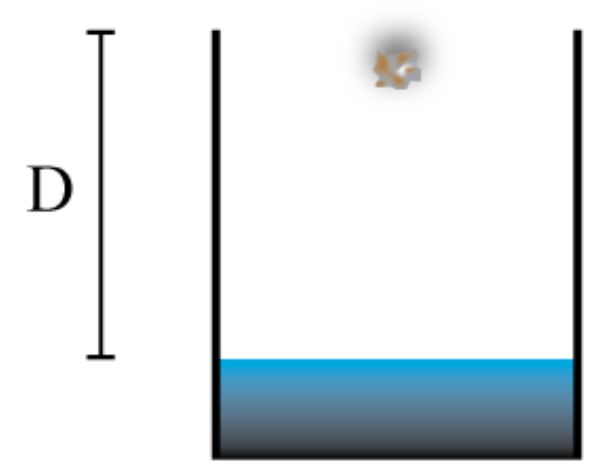# Problem: You stand at the top of a deep well. To determine the depth (D) of the well you drop a rock from the top of the well and listen for the splash as the water hits the water’s surface. The splash arrives t = 4.2 s after you drop the rock. The speed of sound in the well is vs = 331 m/sPart (a) Find the quadratic equation for the distance, D, in terms of the time, the acceleration due to gravity, and the speed of sound. Arrange the expression so that the coefficient of the D2 term is 1.Part (b) Solve the quadratic equation for the depth of the well, D, in meters.

###### FREE Expert Solution

In this problem, we're going to use the equation of motion:

$\overline{){\mathbf{∆}}{\mathbf{y}}{\mathbf{=}}{{\mathbf{v}}}_{\mathbf{0}\mathbf{y}}{\mathbf{t}}{\mathbf{+}}\frac{\mathbf{1}}{\mathbf{2}}{\mathbf{a}}{{\mathbf{t}}}^{{\mathbf{2}}}}$

98% (146 ratings)###### Problem Details

You stand at the top of a deep well. To determine the depth (D) of the well you drop a rock from the top of the well and listen for the splash as the water hits the water’s surface. The splash arrives t = 4.2 s after you drop the rock. The speed of sound in the well is vs = 331 m/sPart (a) Find the quadratic equation for the distance, D, in terms of the time, the acceleration due to gravity, and the speed of sound. Arrange the expression so that the coefficient of the D2 term is 1.

Part (b) Solve the quadratic equation for the depth of the well, D, in meters.